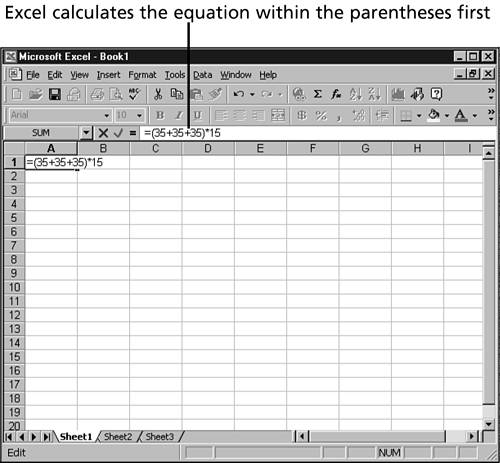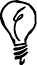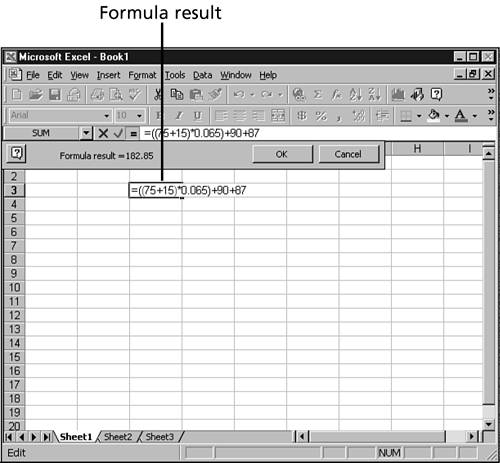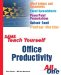# Creating a Simple Formula

Simple formulas that work with values are easy to create. In essence, simple formulas use Excel like a calculator. These simple formulas are somewhat limiting because they don't use any values from the other cells in the worksheet. You can enter a formula into a cell , or you can enter it into the Formula bar.

The following steps give you a chance to create and edit some simple formulas. Because the formulas are only for practice, you should be working on a blank worksheet.

1. The first formula adds a series of numbers together. Click into a blank cell on the worksheet and type =35+35+35 . When you're done typing, press the Enter key. Notice that the result of the calculation, 105 , appears in the cell. The formula is displayed in the Formula bar when the cell is selected.

2. Amend the formula by clicking it and then pressing F2. The formula is displayed in the cell with the cursor flashing at the end of the formula. Type *15 and press Enter.

3. Now edit the formula to add some parentheses. Double-click the cell that contains the formula. The formula appears. Notice that because you're in Edit mode, many toolbar buttons are unavailable. Use the left-arrow keys to move to the first character to the right of the equal sign and then type an opening parenthesis. Use the right-arrow key to move to the last character before the multiplication operator ( * ) and then type a closing parenthesis. When your formula looks like the one in Figure 44.3, press Enter. Notice how the order of calculations changes the result of the calculation.

##### Figure 44.3. The parentheses change the order of operation in the formula.The keystrokes that move you to the beginning and end of a file also work while you're editing a formula. Press Ctrl+Home to quickly move the cursor to the beginning of the formula. Press Ctrl+End to move to the end.

4. Instead of typing formulas directly into a cell, you can enter them into the Formula bar. Select a blank cell and click the Edit Formula button, The Formula palette opens, as shown in Figure 44.4.

##### Figure 44.4. The Formula palette provides a place to enter the formula.5. As you type, the results of the formula are displayed.

6. Click OK when you're done typing. The Formula palette closes , and the results of the formula are displayed in the cell. The formula itself is visible in the Formula bar.You can enter a formula into the Formula bar without using the Formula palette by typing an = sign, rather than clicking the Edit Formula button. When you're done typing, you need to click the green checkmark to enter the formula into the cell.Sams Teach Yourself Office Productivity All in One (Sams Teach Yourself All in One)
ISBN: 0672325349
EAN: 2147483647
Year: 2003
Pages: 474
Authors: Greg Perry